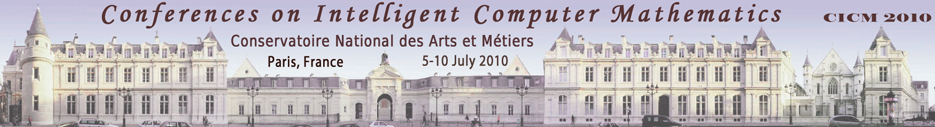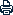## Accepted Papers

The list of accepted papers below is ordered alphabetically by first author surname.

Links to preprints (on http://ArXiv.org) and to the final published articles (on http://www.springerlink.com) will be added as they become available.

The full proceedings of the conference are available from Springer (LNAI 6167). You can find information about it at http://www.springeronline.com/978-3-642-14127-0 or access the online version at http://www.springerlink.com/content/978-3-642-14127-0/

• I-terms in ordered resolution and superposition calculi : retrieving lost completeness
Hicham Bensaid, Ricardo Caferra and Nicolas Peltier
Abstract: Ordered resolution and superposition are the state-of-the-art proof procedures used in saturation-based theorem proving, for non equational and equational clause se ts respectively. In this paper, we present extensions of these calculi that permit one to reason about formulae built from terms with integer exponents (or I-terms), a schematisation language allowing one to denote infinite sequences of iterated terms. We prove that the ordered resolution calculus is still refutationally complete when applied on (non equational) clauses containing I-terms. In the equational case, we prove that the superposition calculus is not complete in the presence of I-terms and we devise a new inference rule, called H-superposition, that restores completeness.

• Structured Formal Development with Quotient Types in Isabelle/HOL
Maksym Bortin and Christoph Lüth
Abstract: General purpose theorem provers provide sophisticated proof methods, but lack some of the advanced structuring mechanisms found in specification languages. This paper builds on previous work extending the theorem prover Isabelle with such mechanisms. A way to build the quotient type over a given base type and an equivalence relation on it, and a generalised notion of folding over quotiented types is given as a formalised high-level step called a design tactic. The core of this paper are four axiomatic theories capturing the design tactic. The applicability is demonstrated by derivations of implementations for finite multisets and finite sets from lists in Isabelle.

• Instantiation of SMT problems modulo Integers
Mnacho Echenim and Nicolas Peltier
Abstract: Many decision procedures for SMT problems rely more or less implicitly on an instantiation of the axioms defining the theories under consideration, and differ by making use of the additional properties of each theory, in order to increase efficiency. We present a new technique for devising complete instantiation schemes on SMT problems over a combination of linear arithmetic with another theory T . The method consists in first instantiating the arithmetic part of the formula, and then getting rid of the remaining variables in the problem by using an instantiation strategy which is complete for T . We provide examples evidencing that not only is this technique generic (in the sense that it applies to a wide range of theories) but it is also efficient, even compared to state-of-the-art instantiation schemes for specific theories.

• On Krawtchouk Transforms
Philip Feinsilver and Rene Schott
Abstract: Krawtchouk polynomials appear in a variety of contexts, most notably as orthogonal polynomials and in coding theory via the Krawtchouk transform. We present an operator calculus formulation of the Krawtchouk transform that is suitable for computer implementation. A positivity result for the Krawtchouk transform is shown. Then our approach is compared with the use of the Krawtchouk transform in coding theory where it appears in MacWilliams' and Delsarte's theorems on weight enumerators.

• A mathematical model of the competition between acquired immunity and virus
Mikhail Kolev
Abstract: A mathematical model describing the interactions between viral infection and acquired immunity is proposed. The model is formulated in terms of a system of partial integro-differential bilinear equations. Results of numerical experiments are presented.

• Some Notes upon "When does <T> equal sat(T)?"
Yongbin Li
Abstract: Given a regular set T in K[x], Lemaire et al. in ISSAC'08 give a nice algebraic property: the regular set T generates its saturated ideal if and only if it is primitive. We dirstly aim at giving a more direct proof of the above result, generalizing the concept of primitivity of polynomials and regular sets and presenting a new result which is equivalent to the above property. On the other hand, based upon correcting an error of the definition of U-set in AISC'06, we further develop some geometric properties of triangular sets. To a certain extent, the relation between the primitivity of T and its U-set is also revealed in this paper.

• How to correctly prune tropical trees
Jean-Vincent Loddo and Luca Saiu
Abstract: We present _tropical games_, a generalization of combinatorial min-max games based on tropical algebras. Our model breaks the traditional symmetry of rational zero-sum games where players have exactly opposed goals (min vs. max), is more widely applicable than min-max and also supports a form of pruning, despite it being less effective than alpha-beta. Actually, min-max games may be seen as particular cases where both the game and its dual are tropical: when the dual of a tropical game is also tropical, the power of alpha-beta is completely recovered. We formally develop the model and prove that the tropical pruning strategy is correct, then conclude by showing how the problem of approximated parsing can be modeled as a tropical game, profiting from pruning.